# Structure of Euclid's Elements

Textual information of definitions, common notions, postulates, and theorems from Euclid’s Elements

## Details

Includes English and Greek text of statements with word count for definitions, common notions, postulates and theorems, with English and Greek text and reference of proofs for theorems.

## Examples

### Basic Examples

Find all information of Book 1, Theorem 20:

 In:=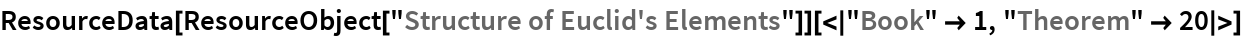Out=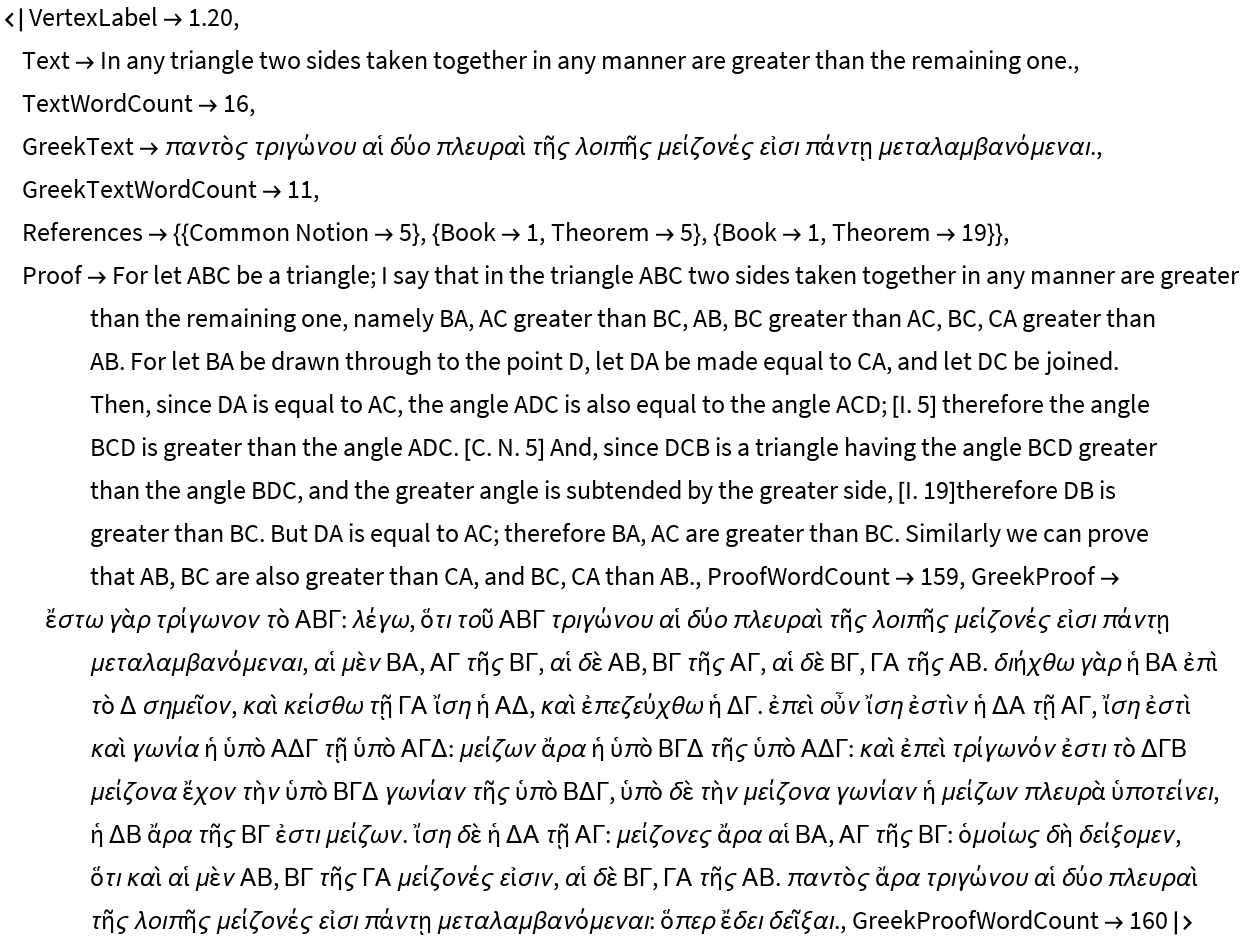Find specific information of Book 1, Theorem 20:

 In:=Out=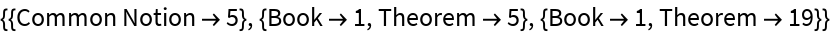### Analysis

Find the axioms:

 In:=Out=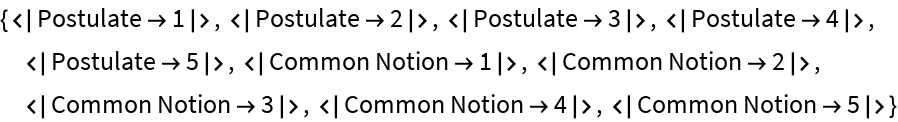Find the definitions of Book 3:

 In:=Out=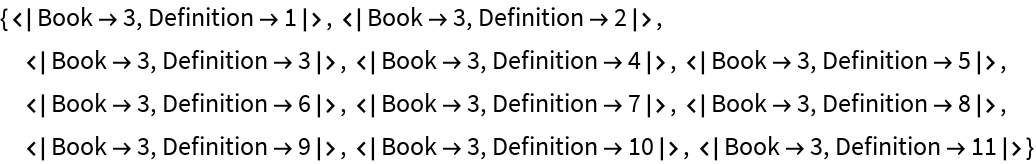Find the theorems of Book 4:

 In:=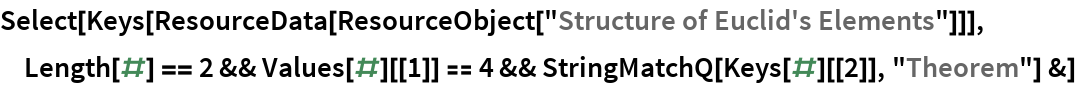Out=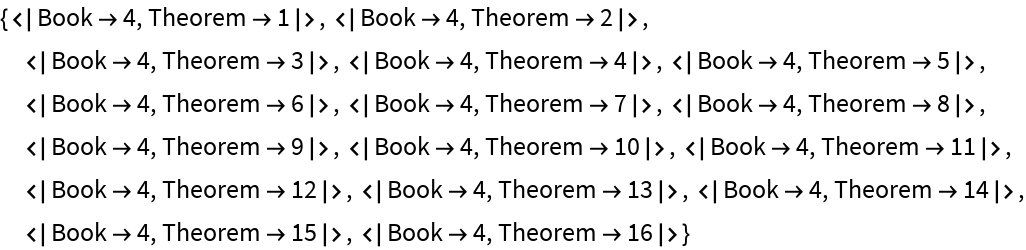Count the total number of theorems:

 In:=Out=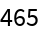Wolfram Research, "Structure of Euclid's Elements" from the Wolfram Data Repository (2020)